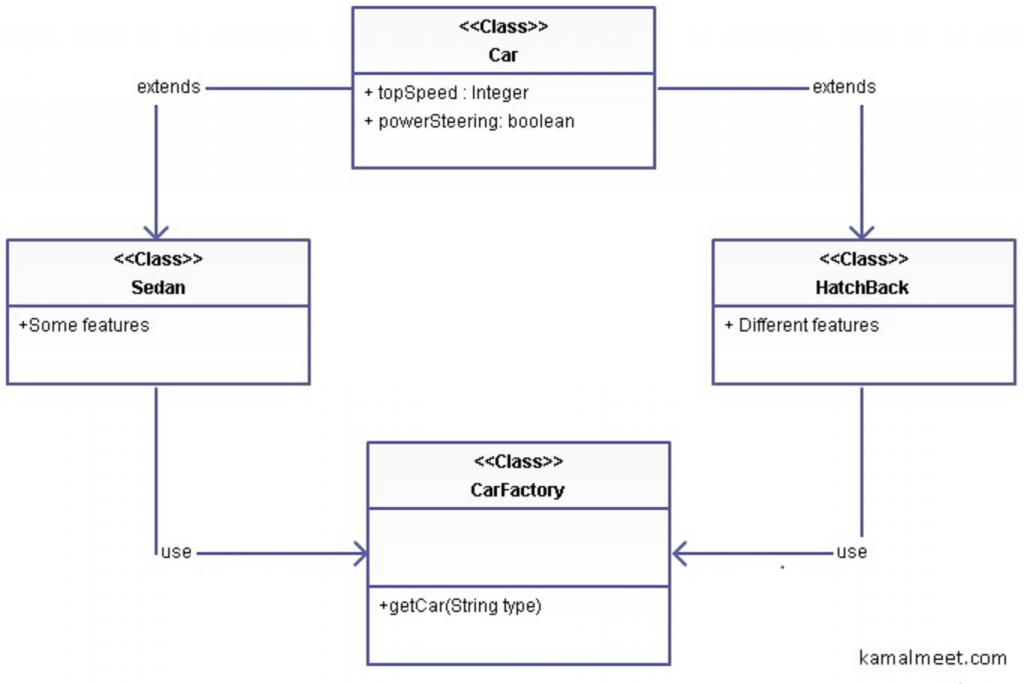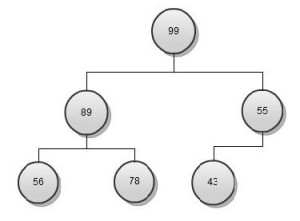# Interesting Java Facts 3: Cloning a multidimensional Array

Consider the following code

int arr[][]={{10,20,30},{23, 45, 67,}};
int clone[][]=arr.clone();
clone=-1;

Q. What should be the value of arr and clone ?

A. It is -1 for both. Why? Let understand 2 things- clone always make a shallow copy of object  & Array is an object in Java.

Shallow copy: When we clone an object in Java, it only creates duplicate copies of primitive types, and for objects, the reference is copied. For example if an Employee object containing an address object is cloned, for address only reference is copied, that is not bot employee and clone are referencing same address object and any change in address is reflected in both.

We can override the clone method for Employee to make sure whenever a clone is made a new object for Address is created as well and all the values are actually copied one by one to new object. This will make sure both the original object and clone are referencing to their own address and changes in one does not get reflected in other.

Array is an object: In java array is an object, so a multidimensional array is actually an object with multiple objects (arrays) in it.

Putting both the above concepts together, we can figure out that while cloning, only the references of array objects  in multidimensional array are copied to cloned object, that means any change in one will get reflected to another.

# Revisiting Max Subarray Problem

Sometime back I discussed and solved a problem to find out a subarray from an array which has the maximum sum- here.

I solved the problem using brute force, that is, all the sub arrays were actually created and maximum sum subarray was found. That solution was created in order of N^2 complexity.

Let’s see how can we improve on our solution using divide and conquer approach.

Array: {-1,2,4,60,1,3,-2,-6,7,-9}

If we divide the original array into two half arrays from mid of the array. one of the following must be true

1. Max Subarray is part of first half {-1,2,4,60,1}

2. Max Sub array is part of second half {3,2,-6,7,-9}

3. Max Subarray passes through the mid of array

Array with maximum sum and passes through mid can be easily figured out in order of N. In this we will simply keep moving left and right from the mid until we hit maximum sum array, so first subarray would be 2+4+60+1+3=70

To solve 1 and 2 we will recursively use the approach with the 2 sub arrays (Repeatedly divide the array and find max having mid of the array).

The problem set is getting reduced to half with every iteration, so Log N iterations will be there in total. At max N elements are added (compared to be -ve), in each iteration, hence the total complexity of the algorithm is (N log N), so we are able to reduce the complexity from N^2 to (N log N) with the help of divide and conquer approach.

# Merge Sort: Divide and Conquer Approach

Merge sort is classic example of divide and conquer algorithm approach.

Divide and Conquer Algorithm Design approach: The idea is simple, as the term suggest, we try to divide the original problem into multiple smaller problems. These small problems are solved and the solutions then are merged into one final solution.

The concept is used in merge sorting. Input to any sorting algorithm is a random list (array). For sake of this example let’s take a random array of numbers as input.

Divide Phase: Divide the main array into smaller arrays upto a level that it can sorted easily.

Conquer: Merge these entire sorted arrays into the final solution array.

Example – Lets say we have an array

Original: 99, 23, 45, 12, 67, 28, 09, 98, 44, 84

Divide Phase

Arr1: 99, 23, 45, 12, 67                | Arr2:   28, 09, 98, 44, 84

99, 23, 45                    | 12, 67        |28, 09, 98              | 44, 84

99, 23        | 45           |12    | 67     |28, 09        | 98      |44     | 84

99   | 23   | 45           |12    | 67       |28    | 09   | 98      |44     | 84

Merge Phase

23, 99      | 45           |12, 67            |09, 28   | 98           | 44, 84

23, 45, 99                  | 12, 67          | 09, 28, 98              |   44, 84

12, 23, 45, 67, 99                            | 09, 28, 44, 84, 98

09, 12, 23, 28, 44, 45, 67, 84, 98, 99

Complexity Analysis

The Divide phase of merge sort will need log N operations as in every step we are reducing length of array by half.

Merge step will again have log N merges and in each merge step we will have maximum N comparison, so the overall complexity will (N log N)

# Factory Pattern

Factory Pattern is one of the highly used design patterns. The reason being , its genuine usefulness and closeness to real world. Let’s take a very simple real world example to get started, and then we will map it to the pattern.

Let’s say we have a Car factory which creates many types of cars. To keep it simple, let’s say it creates 2 type of cars- hatchback & sedan. These two type of cars will have many similar attributes like top speed, power steering etc. but there will be some features explicit to type of car (say a back seat AC or TV is available only for sedan cars). What will be a natural Object Oriented Design for this arrangement?

Class Car Extended by Class Sedan and Class HatchBack

If you know what type of car you need beforehand, we are good. But in case you need to create the car at runtime, say on user input, you will need some helper class which will create specific type of car at runtime. This class is our factory class.Class CarFactory

{

Public static Car getCar(String carType){

If(carType.equals(“sedan”)) return new Sedan();

else return new HatchBack();

}

}

A better example in technical terms might be that your application supports multiple database types (say on test environment you use MySql whereas on production you have Oracle). A DataBaseConnectionFactory can help you get connection of specific type of database at runtime (say reading the database type value from some property file.

# Heapsort: Algorithm and Complexity

Heap sort is one of the most important and interesting algorithms. The implementation is base of many other sophisticated algorithms. We will focus on basics of heap sorting here. Infact, if you understand what a heap data structure is, heap sort is just application of some known simple operations on the heap.

Let’s relook at our heapThis is a max heap so let’s try to create an array in reverse sorted order (a min heap is good for sorted in lowest to highest format and vice versa).  Before moving forward, revisit the heap data structure and understand Insert and Delete operations, after that heap sort algorithm is a cake walk.

We know that max heap has a property that element at the top is highest. Using this property, we can create an algorithm like

1. Create max heap from the random array
2. Remove top element from heap and add to reverse sorted array
3. Heapify (restore heap property) the remaining heap elements
4. Repeat Step 2 till the heap is empty

Step 1 is nothing but inserting all elements of an array to heap. One Insert Key operation takes Log N operations so N elements will take N Log N

Step 2 is order of 1 as we are just removing the top element and adding to our array

Step 3 is nothing but Delete Node operation in heap, i.e.  take last leaf node and add as root, then compare with its child nodes and swap with largest. Repeat the process till heap property is maintained, i.e. child is greater than parent.  This is Log N operations for one heapify.

Step 4 is combination of 2 & 3, N times. Step 2 is constant order and hence can be ignored. This means we have N Step 3 order or N Log N.

Combining Step 1 and 4, we figure out order of heap sort complexity is of  N Log N order.

http://kamalmeet.com/2011/07/insertion-sorting/

# Java multiple Inheritance: Interfaces and Composition

Inheritance means a class extending other class. Multiple Inheritance means single class extending more than one class. Java does not support this kind of inheritance as one class can inherit only a single class.

Q. Why Java does not support multiple inheritance?

Consider a scenario, there are 2 classes

class A{

public void mymethod(){//some code

}

}

class B{

public void mymethod(){//some code

}

}

class C extends A,B{//illegal in Java

//which implementation of mymethod is imported ?

}

If java allow multiple inheritance, it will be tricky to handle above situation with multiple inheritance, hence Java does not allow it.

Q. How does Java implement Multiple inheritance?

Java does not provide direct implementation of multiple inheritance by extending more than one classes, but it provides you 2 alternatives.

1. Use of Interfaces: A Java class cannot extend more than one class, but it can implement any number of interfaces.

Class C implements A,B{ // this time A and B are interface

public void mymethod(){//interfaces did not provide implementation and hence no conflict

}

}

2. Composition: Other way to use properties of other classes is to use composition, that is have the object of other classes as part of current class.

Class C{//A and B are different classes

A objA=new A();

B objB=new B();

}

Best Approach: A mix of implementing interfaces, extending class and using composition, based on different requirements